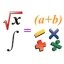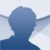# GEUP

9.0.1.0

## GEUP is a complete maths environment to carry out calculations and graphically represent problems. Download GEUP to have a better understanding of maths

Certain Mathematics fields require a good dose of spatial interpretation. That happens for example with Geometry, subject in which it's usual to use, as well as calculations, graphical representations to find out the solution to the problem that has been posed.

To solve all kinds of problems (algebraic, geometric, etc.) that require carrying out calculations and graphic representations, we have GEUP, a powerful real-time mathematical environment, that makes the studying of Mathematics and its multiple fields a lot easier.

### Main features

• Calculations and graphic representations of functions.
• Support to open several documents and work with different coordinate systems at the same time.
• Use of macros.
• Implementation of all sorts of geometric transformations.
• Visualization of geometrical intersections and animations with speed control.
• Printing options.
• Totally customizable application.

If you need help to understand Mathematics, try out GEUP now!